• V1.21
Examples
• 19 Dec 2022
• PDF

# Examples

• PDF

## Reading tag and timestamp values from a MySQL database

In this example, SQL Client is used to connect to a MySQL database located in the same machine in order to extract the following data: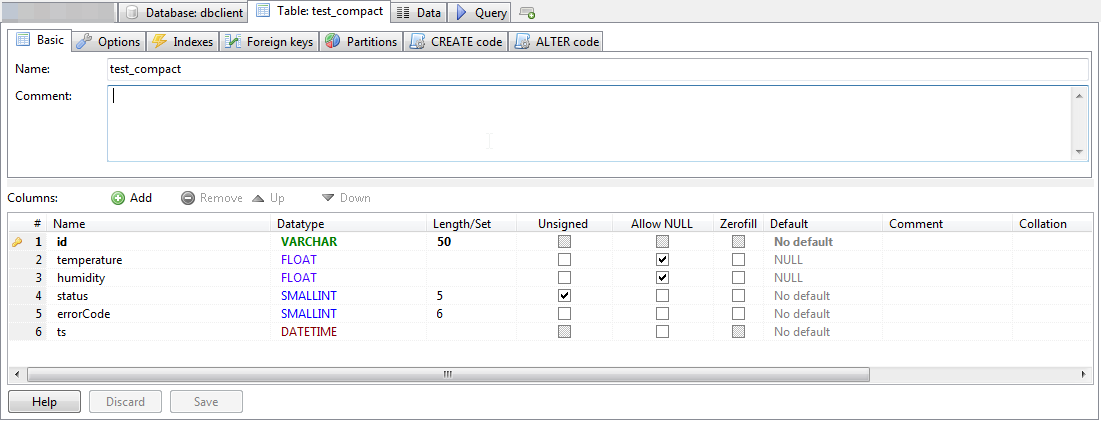Figure 14 Table structure in the database

• Step 1: Configuration of the connection.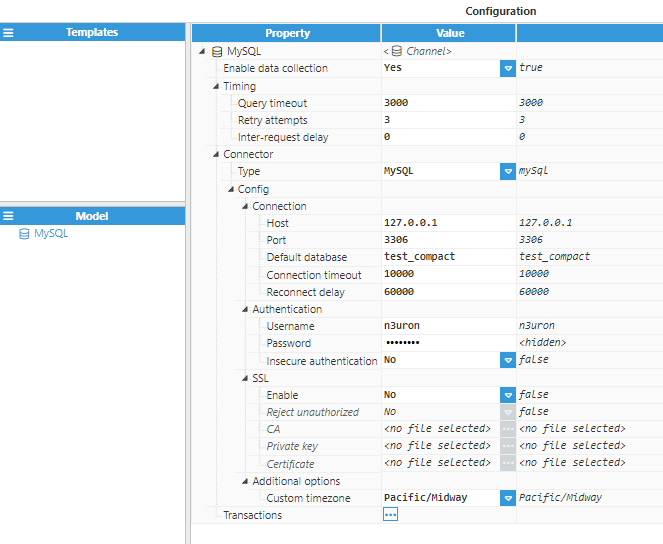Figure 15: Configuring the connection to the MySQL database

• Step 2: Configuration of the transaction.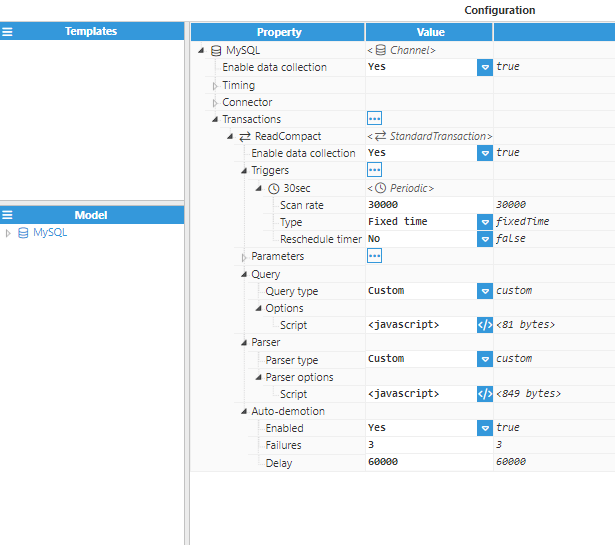Figure 16: Configuring the transaction

The Query script for requesting the required columns from the data base:

``\$.output = "SELECT id, temperature, humidity, status, errorCode, ts FROM test_compact";``

The Parser script iterates through the Dataset returned from the query, starting by iterating all rows (one row per sensor) and then iterating through the columns for each individual row (one column per signal):

``````for(const row of \$.input.rows()){
//Iterate through the columns of the Dataset for each row
for(const col of \$.input.columns()){
//Get the name of the current column
const column = col.getName();
\$.logger.debug("Column is %s", column);
//Ignore id and ts columns
if(column === "id" || column === "ts") continue;
const tagData = {};
//Set the destination tag using the format rowid_columnName
tagData.tag = row.getValue("id")+"_"+column;
//Get the value of the current column at this row
tagData.value = row.getValue(column);
//Set quality to 192
tagData.quality = 192;
//Set the ts to the value of the ts column
tagData.ts = row.getValue("ts");
\$.logger.debug("Tag data is %j", tagData);
//Push the tag data object to the output array
\$.output.push(tagData);
}
}
``````

• Step 3: Tag configuration settings for linking the data received from the database to tags.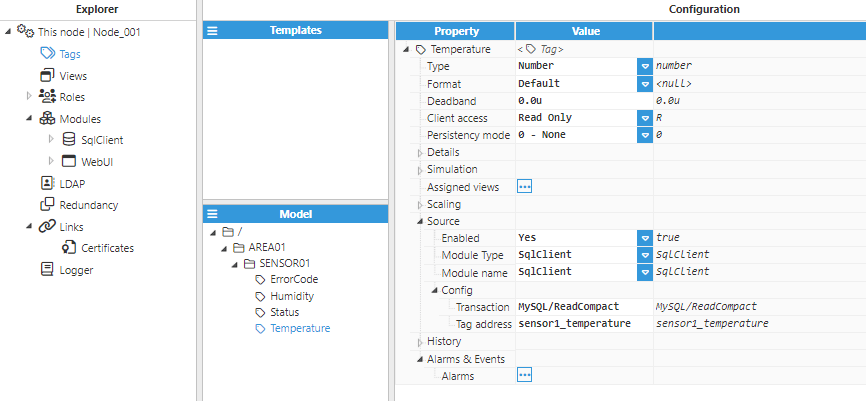Figure 17: Configuration of any tags updated with values received from the database

## Inserting historical data into a database

This example explains how to use a HistoricalTransaction to insert N3uron tag events into a database.

The database in this example is MySQL and as such, a syntax that is compatible with MySQL is used.

• Step 1: The target table should have the following schema: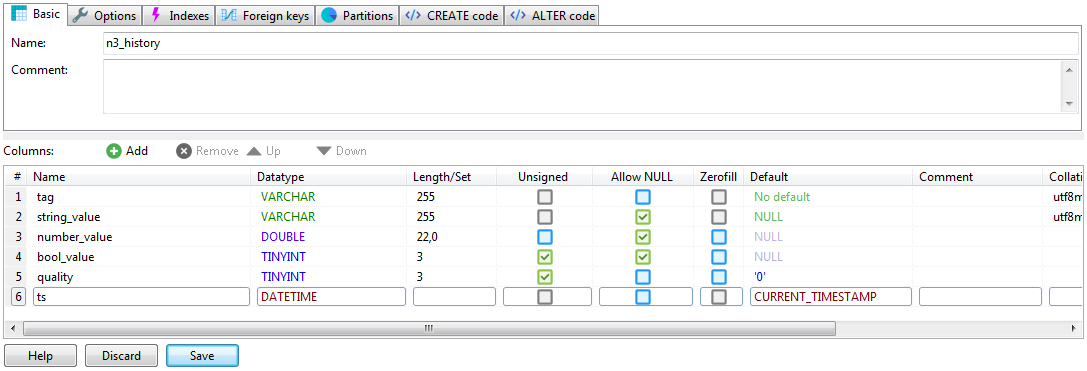Figure 18: MySQL table schema

• Step 2: The following screenshot shows the HistoryTransaction configuration: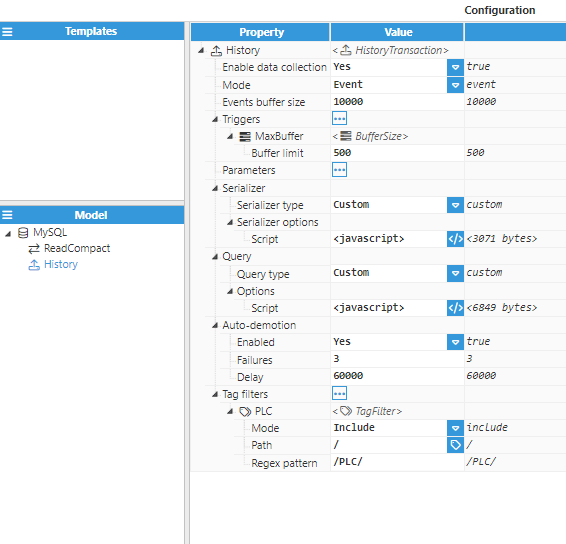Figure 19: HistoryTransaction configuration

• Step 3: The following data serialization script is used:
``````const ds = new Dataset();
//Add all columns that are used to the Dataset
for (const event of \$.input) {
const row = {
//Tag is escaped with the string separator for MySQL
tag: "'"+event.tag+"'",
string_value: null,
number_value: null,
bool_value: null,
quality: event.quality,
ts: event.ts
}
//Depending on the type of the event, set the relevant field
switch (typeof event.value) {
case "number":
row.number_value = event.value;
break;
case "string":
//Add string quotes since string values need to be quoted in the query
row.string_value = sprintf("'%s'", event.value);
break;
case "boolean":
row.bool_value = event.value;
break;
}
//Add the row to the Dataset
}

//If the output row count is 0, return empty Dataset
if (ds.getRowCount() === 0) \$.output = new Dataset();
else \$.output = ds;
``````

• Step 4: The next query builder is used:
``````//If the input Datasets has no rows, return an empty string to abort the query
if(\$.input.getRowCount() === 0){
\$.output = ""
} else {
\$.output += _insertData();
}

function _insertData(){
const values = [];
//Iterate through all the rows in the input Dataset
for(const row of \$.input){
//String variable that holds the values to insert for each row
let rowValues = "(";
rowValues += row.getValue("tag")+",";
rowValues += row.getValue("string_value")+",";
rowValues += row.getValue("number_value")+",";
rowValues += row.getValue("bool_value")+",";
rowValues += row.getValue("quality")+",";
//Timestamp is created using the MySQL function FROM_UNIXTIME(epoch).
rowValues += sprintf("FROM_UNIXTIME(%d)", row.getValue("ts").getTime()/1000);
rowValues += ")";
//Push the row values into the values array
values.push(rowValues);
}
//Return the query
const query = sprintf("INSERT INTO n3_history (tag, string_value, number_value, bool_value, quality, ts) VALUES "+ values.join(",\r\n"));
return query;
}
``````

This query will execute in event mode (it only sends new tag events) and the trigger will be a buffer size trigger, which means that the transaction triggers whenever there are 500 events or more in the buffer.

## Creating INSERT queries from parameters

This example deals with a common scenario whereby a number of tag values need to be periodically inserted into the database. In this example, a StandardTransaction is used, since this transaction is recommended for these types of queries. The database connection is assumed to be already created and working.

The syntax used in this example is MySQL. If the destination database is different, the syntax might require small changes within the query.

• Step 1: Configuration of the transaction.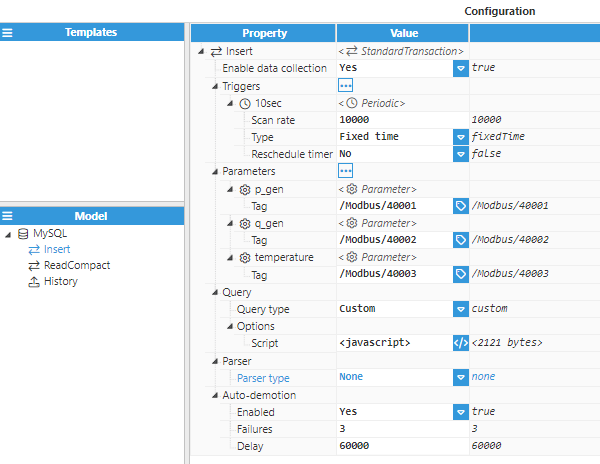Figure 20: StandardTransaction configuration

• Step 2: Script used to create the query.
``````const TABLE_NAME = "n3_data";

\$.output = insert();

function insert(){
const query = sprintf("INSERT INTO %s (%s) VALUES (%s)", TABLE_NAME, getColumns().join(","), getValues().join(","));
return query;
}

function getColumns(){
const columns = [];
//Iterate through all the parameters
for(const p of Object.keys(\$.parameter)){
//Use the parameter name as column name, and escape it using the escape character `
columns.push(sprintf("`%s`", p));
}
return columns;
}

function getValues(){
const values = [];
//Iterate through all the parameters
for(const value of Object.values(\$.parameter)){
//Cast the value into the appropiate type and push it into the values array
values.push(cast(value));
}
return values;
}
function cast(value){
//If the value is null, the string NULL is returned
if(value === null){
return "NULL";
}
switch (typeof value){
case "number":
//If the number is value, return it without changing
return value;
case "string":
//If the value is a string, convert it to a MySQL string using '
return "'"+value+"'";
case "boolean":
//If the value is a boolean, convert it to a 0 or 1 number
return Number(value);
default:
//If the type is none of the above, throw an error since
//only the above types are supported in this example
throw new Error("Unknown type for value: "+typeof value);
}
}
``````

## Synchronising data from N3uron to database

In this example, data from N3uron is going to be synchronised with the database, so that both N3uron and the database contain the same data. The tags that will be synchronised come from an external device (whether this be a PLC, a Modbus device, or an OPC UA Server) and are set up as parameters in the transaction.

This example uses a StandardTransaction, since this is the ideal transaction for this scenario.

The destination database in this example is MySQL and as such, the syntax for MySQL is used.

• Step 1: The database destination table has the following schema: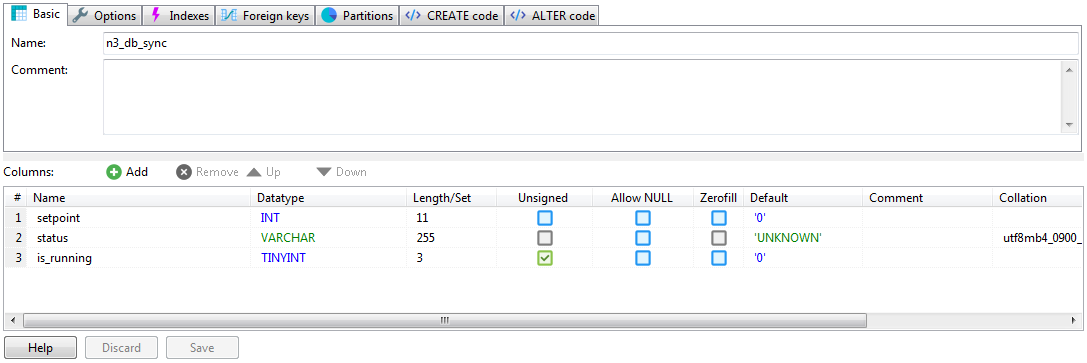Figure 21: MySQL table schema

• Step 2: Configuration settings for StandardTransaction: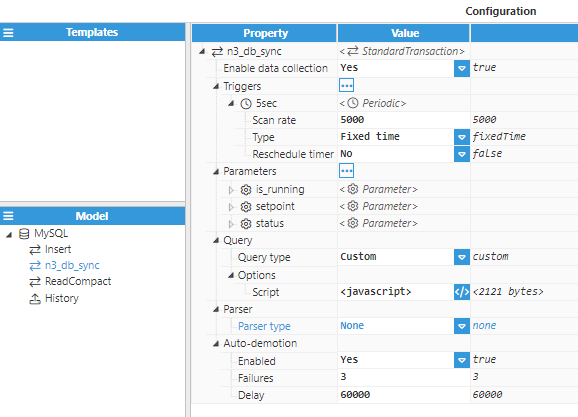Figure 22: StandardTransaction configuration

• Step 3: Script used to create the query:
``````\$.output = sprintf("UPDATE `n3_db_sync` SET `setpoint`=%f, `status`='%s', `is_running`=%d",
\$.parameter.setpoint.value,
\$.parameter.status.value,
\$.parameter.is_running.value);
``````

This query will be executed every 5 seconds, and will update the "n3_db_sync" table with the current values in N3uron. This table has a single row, which contains the values that were synchronised between N3uron and the database.

## Synchronising data from the database to N3uron

This example is analogous to the previous example, except that the data is synchronised in the opposite direction, from a database to N3uron tags.

The destination tags in this example are not SQL Client source tags, instead, they are tags that are driven by other modules, such as Modbus, Siemens S7, etc. This is done by using a StandardTransaction, since this transaction supports writes to tags. These tags must also have Read/Write permissions, otherwise the write will fail

The destination database is MySQL, so the MySQL syntax is being used.

• Step 1: The destination table has the following schema: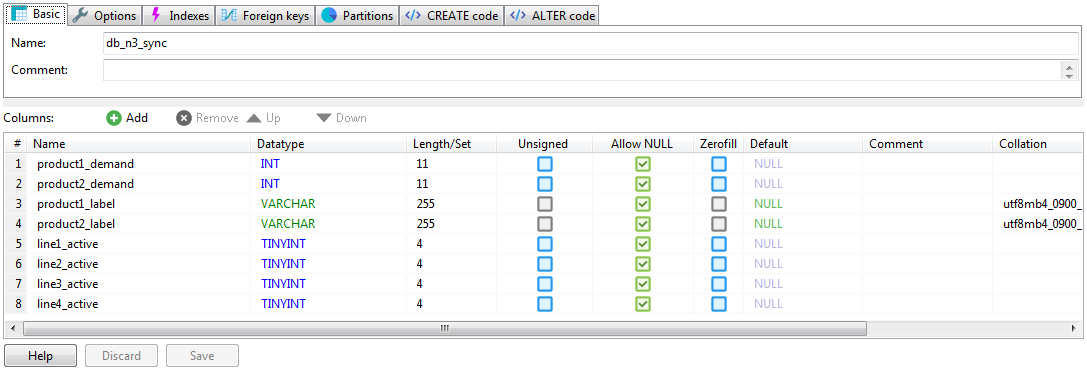Figure 23: MySQL table schema

• Step 2: The following StandardTransaction configuration is used: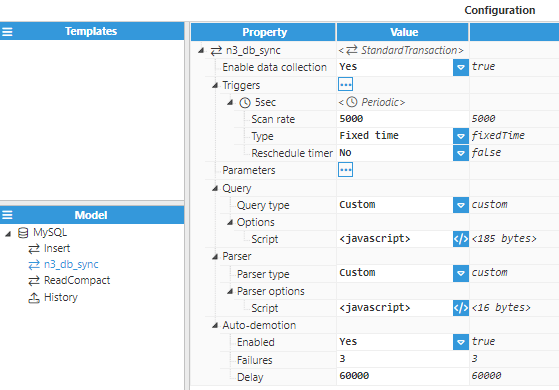Figure 24: StandardTransaction configuration

• Step 3: The query script is as follows:
``\$.output = "SELECT `product1_demand`, `product2_demand`, `product1_label`, `product2_label`, `line1_active`, `line2_active`, `line3_active`, `line4_active` FROM db_n3_sync";``

• Step 4: The parser script is as follows:
``````\$.output.push({tag:"/PLC/product1_demand",value:\$.input.getValue(0, "product1_demand")});
\$.output.push({tag:"/PLC/product2_demand",value:\$.input.getValue(0, "product2_demand")});
\$.output.push({tag:"/PLC/product1_label",value:\$.input.getValue(0, "product1_label")});
\$.output.push({tag:"/PLC/product2_label",value:\$.input.getValue(0, "product2_label")});
\$.output.push({tag:"/PLC/line1_active", value: \$.input.getValue(0, "line1_active")});
\$.output.push({tag:"/PLC/line2_active", value: \$.input.getValue(0, "line2_active")});
\$.output.push({tag:"/PLC/line3_active", value: \$.input.getValue(0, "line3_active")});
\$.output.push({tag:"/PLC/line4_active", value: \$.input.getValue(0, "line4_active")});
``````

This transaction will be executed every 5 seconds and will write all data obtained in the first row of the query to the specified tags.